Next: Non-linear cross-correlations Up: Multi-unit contrast functions Previous: Likelihood and network entropy

### Mutual information and Kullback-Leibler divergence

Theoretically the most satisfying contrast function in the multi-unit case is, in our view, mutual information.

Using the concept of differential entropy defined in Eq. (6), one defines the mutual information I between m (scalar) random variables yi, i=1...m, as follows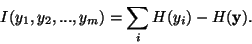(15)

where H denotes differential entropy. The mutual information is a natural measure of the dependence between random variables. It is always non-negative, and zero if and only if the variables are statistically independent. Thus the mutual information takes into account the whole dependence structure of the variables. Finding a transform that minimizes the mutual information between the components si is a very natural way of estimating the ICA model . This approach gives at the same time a method of performing ICA according to the general Definition 1 in Section 3. For future use, note that by the properties of mutual information, we have for an invertible linear transformation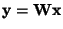: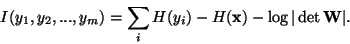(16)

The use of mutual information can also be motivated using the Kullback-Leibler divergence, defined for two probability densities f1 and f2 as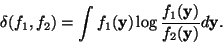(17)

The Kullback-Leibler divergence can be considered as a kind of a distance between the two probability densities, though it is not a real distance measure because it is not symmetric. Now, if the yi in (15) were independent, their joint probability density could be factorized as in the definition of independence in Eq. (7). Thus one might measure the independence of the yi as the Kullback-Leibler divergence between the real density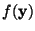and the factorized density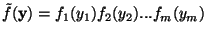, where the fi(.) are the marginal densities of the yi. In fact, this quantity equals the mutual information of the yi.

The connection to the Kullback-Leibler divergence also shows the close connection between minimizing mutual information and maximizing likelihood. In fact, the likelihood can be represented as a Kullback-Leibler distance between the observed density and the factorized density assumed in the model . So both of these methods are minimizing the Kullback-Leibler distance between the observed density and a factorized density; actually the two factorized densities are asymptotically equivalent, if the density is accurately estimated as part of the ML estimation method.

The problem with mutual information is that it is difficult to estimate. As was mentioned in Section 2, to use the definition of entropy, one needs an estimate of the density. This problem has severely restricted the use of mutual information in ICA estimation. Some authors have used approximations of mutual information based on polynomial density expansions [36,1], which lead to the use of higher-order cumulants (for definition of cumulants, see Appendix A).

The polynomial density expansions are related to the Taylor expansion. They give an approximation of a probability density f(.) of a scalar random variable y using its higher-order cumulants. For example, the first terms of the Edgeworth expansion give, for a scalar random variable y of zero mean and unit variance :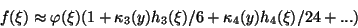(18)

where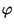is the density function of a standardized Gaussian random variable, the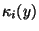are the cumulants of the random variable y (see Appendix A), and hi(.) are certain polynomial functions (Hermite polynomials). Using such expansions, one obtains for example the following approximation for mutual information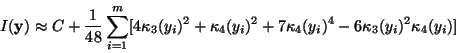(19)

where C is constant; the yi are here constrained to be uncorrelated. A very similar approximation was derived in , and also earlier in the context of projection pursuit in .

Cumulant-based approximations such as the one in (19) simplify the use of mutual information considerably. The approximation is valid, however, only when f(.) is not far from the Gaussian density function, and may produce poor results when this is not the case. More sophisticated approximations of mutual information can be constructed by using the approximations of differential entropy that were introduced in , based on the maximum entropy principle. In these approximations, the cumulants are replaced by more general measures of nongaussianity, see Section 4.4.3 and Section 4.4.1. Minimization of such an approximation of mutual information was introduced in .Next: Non-linear cross-correlations Up: Multi-unit contrast functions Previous: Likelihood and network entropy
Aapo Hyvarinen
1999-04-23